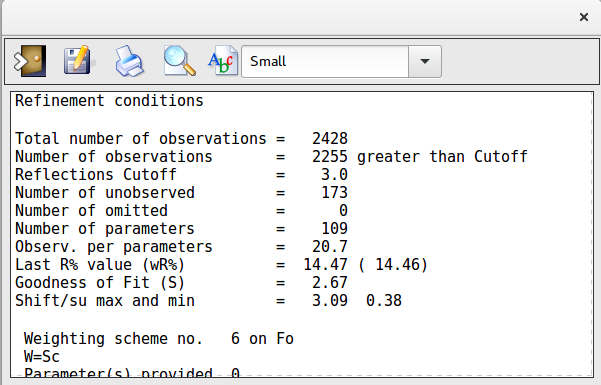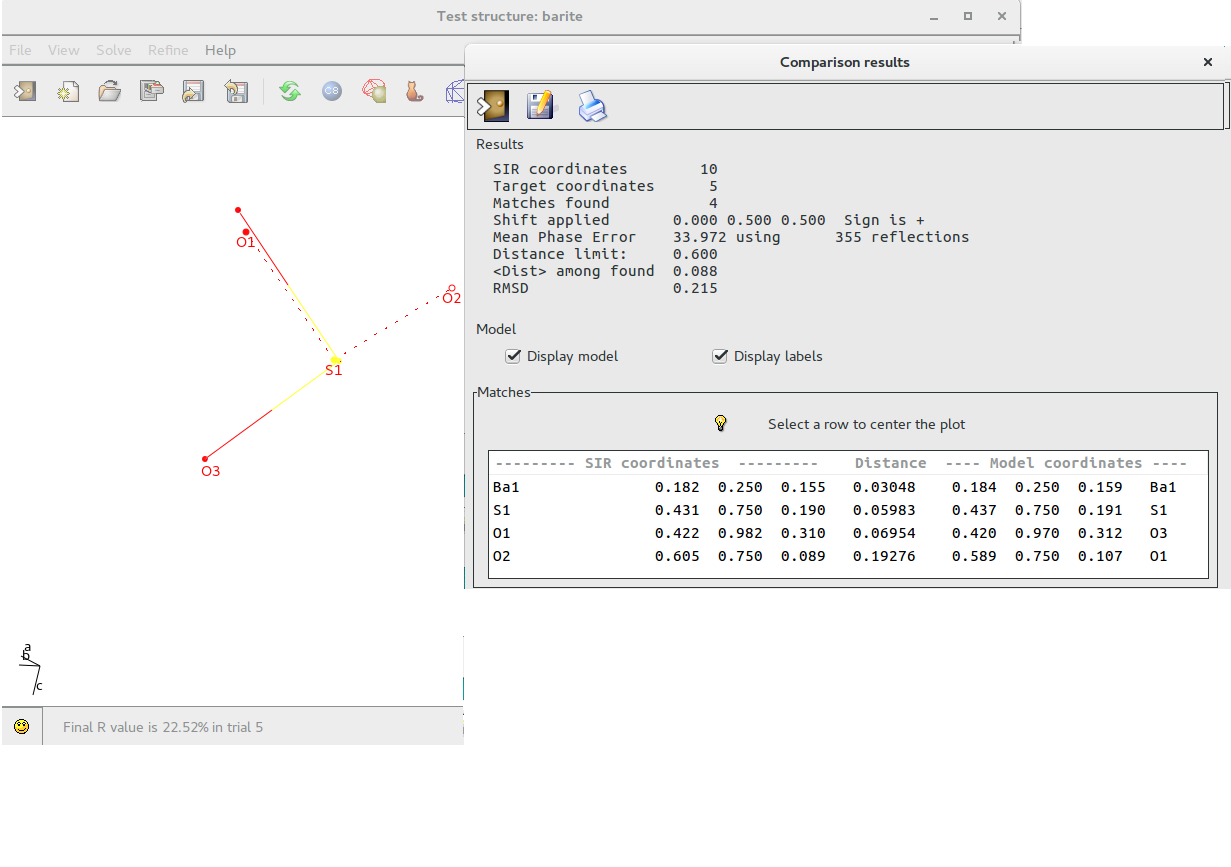# RefineIt allows:LSQLSQ infoThermal FactorFourierCompare Atoms

LSQ

Least Squares graphical interface is divided in 2 sections:

• General
• Modify Atoms Parameters

The program provides default values but the user can change them.

In the first section the user defines the Least Squares strategy (Diagonal Matrix, Full Matrix or Structure Factor Calculation only) and the Refinement Conditions such as Damping Factor, Reflections cutoff, number of cycles. 18 Weighting Schemes are available and their parameters can be modified. At the bottom of this window information about the ratio Observations/Parameters is updated depending on user choices.

To start the calculations click onTo get help click onIn this section the user can fix or relax the atom parameters (coordinates, thermal factor) and set the thermal factor isotropic or anisotropic.LSQ info

Information about Least Squares calculations such as atom coordinates, isotropic/anisotropic thermal factor, conditions on atom parameters (fixed, equivalence,..), last value of the residual are displayed.Thermal Factor

During Least Squares calculations the value of the thermal factor for some atoms can assume too large values. This window can be used to analyse the values of the thermal factor and, eventually, delete the atoms whose value is too high.Fourier

In order to locate new atoms, a Fourier interface is available.

Once the Fourier type has been selected and the number of new peaks has been decided, the calculations are started by clicking onbutton.

To get help click on###Fourier type

• Observed: The coefficients of the Fourier synthesis are the observed structure factors (Fobs).
• 2*Fobs Fcalc: The coefficients are two times the Fobs minus the calculated structure factors (Fcalc).
• Fobs Fcalc: The coefficients are the difference between the observed and the calculated structure factors.

### Fourier Conditions

• Peaks to add: The user can select the maximum number of peaks to be located.
• Level (electrons/Å<sp&nbspan class=”rvts106″>3): The peaks to be located must have the height greater than this value of the electron density.

### Fourier Space

• Unit Cell: The asymmetric part defined by the Space Group is the space where the Fourier synthesis has to be calculated.
• Cube: The user can define a cube, having the faces parallel to the cell faces, where the Fourier synthesis has to be calculated. The dimension in Angstrom of the cube edge (default 2.0 Å) and the grid stepof the Fourier synthesis (default 0.20 Å) can be defined by the user. Using the mouse and moving the model, the user can place the cube where it is needed.

If View Fourier map is checked, the Fourier map and the model are shown by  Jav .

The peak search routine provides a number of new peaks. Information about peak intensity and distance from the closest atom is provided by means of the window “Select peaks to accept”, so the user can select peaks to retain. New peaks (two in this example) are coloured in light blue in the Jav window.By means of the Jav interface is possible to delete them or to assign an atomic species.

Compare Atoms

If a structural model is available (i.e. solving a structure using electron diffraction data an X-Ray model could be available), it is possible to compare the coordinates provided by the program with the model.

Using the following window it is possible to load the model to compare:The result of the comparison is shown below; the model is superimposed and drawn using dotted lines. The list of the atoms matching the coordinates of the reference model is produced.